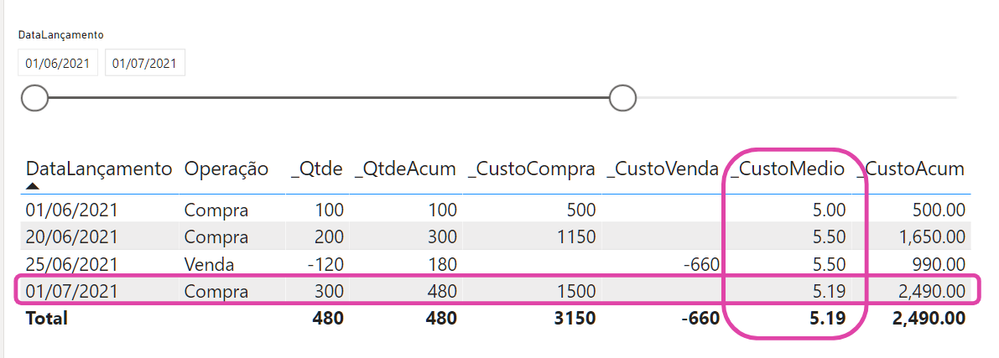cancel
Showing results for
Did you mean:Anonymous
Not applicable

## Weighted Moving Average

```Weighted Moving Average

Hello.

Personal, my doubt is how to calculate the weighted moving average, in Power Bi, the problem is when I have to calculate the cost of the unit sold, multiplying by the average cost, and deducting this value from the Total.

Initial = 100 Units of Product X, at the cost of R\$ 500.00 per batch

Acquisition of 200 Units of Product X, at the cost of R\$ 1,150.00 per lot

The average cost of Product X will be:

BRL 500.00 + BRL 1,150.00 divided by (100 + 200) units

= BRL 1,650.00: 300

= BRL 5.50 per unit

In the effective sequence to a write-off of -120 units

= 120 multiplied at the cost of R\$ 5.50 = -660

Accumulated cost goes from R\$ 1650.00 - 660 = 990 and the Total of 300 units - 120 = 180, maintaining the average cost, until a new acquisition.

In the example below, where it is 7.19 the correct is 5.81, which would be R\$ 1650.00 - 660.00 +1800 = 5.81, if there is no sale Works perfectly.```6 REPLIES 6Solution Sage

Your visual does not look like the one you've shown above:Anonymous
Not applicableSolution Sage

I don't understand now... You've improved the formula, so it is already working fine?Anonymous
Not applicable

No, it's still wrong, note in the image I updated, the correct result is from excel.Anonymous
Not applicable
`Can anyone help me with this question?`Solution Sage

@Anonymous

Would you please format the post in a convenient way to read it? Currently it requires people to scroll across and is not very readable. The font is weird as well. Thanks.